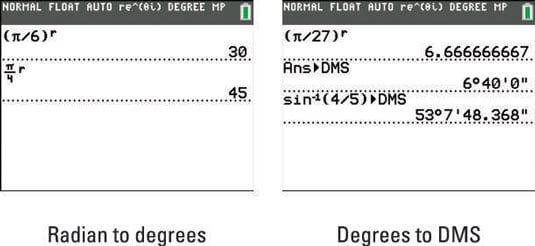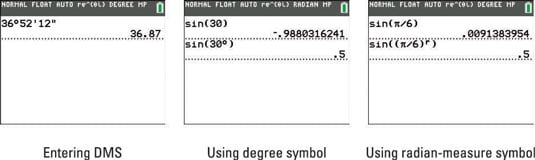##### TI-84 Plus CE Graphing Calculator For Dummies, 3rd EditionThe functions available in the TI-84 Plus calculator's Angle menu enable you to convert between decimal degrees and DMS (degrees, minutes, and seconds). You can also override the angle setting in the Mode menu of the calculator when you use these functions.

To convert decimal degrees to DMS (degrees, minutes, and seconds), follow these steps:

1. Put the calculator in Degree mode.

Press [MODE], use the arrow keys to highlight DEGREE, and then press [ENTER].

2. If necessary, press [2nd][MODE] to access the Home screen.

3. Enter the degree measure.

4. Press [2nd][APPS][ENTER] to convert the degrees to DMS.

This is illustrated in the second screen.## Entering angles in DMS measure

To enter an angle in DMS measure (and convert to decimal degrees), follow these steps:

1. Enter the number of degrees and press [2nd][APPS] to insert the degree symbol.

2. Enter the number of minutes and press [2nd][APPS] to insert the symbol for minutes.

3. Enter the number of seconds and press [ALPHA][+] to insert the symbol for seconds.

4. Press [ENTER] to evaluate your DMS measure.

Since your calculator is in Degree, pressing [ENTER] converts DMS to decimal degrees. See the first screen.## Overriding the mode of the angle

If the calculator is in Radian mode but you want to enter an angle in degrees, enter the number of degrees and then press [2nd][APPS] to insert the degree symbol. Essentially, you’re forcing your calculator to evaluate your angle in degrees regardless of the mode setting. Getting into the habit of adding the degree symbol to your angle gives your math teacher the warm fuzzies all over!

If the calculator is in Degree mode and you want to enter an angle in radian measure, enter the number of radians and then press [2nd][APPS] to insert the radian-measure symbol.

If the radian measure is entered as an arithmetical expression, be sure to surround it with parentheses before you insert the radian-measure symbol!